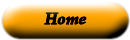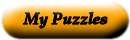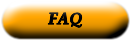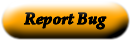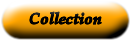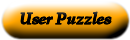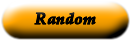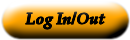### Sciecne

madelyn

 Centripetal acceleration the product of its mass and velocity Centripetal force if the net force acting on an object is zero the object remains at rest First law of motion if a group of objects exerts forces only on each other their momentum doesn't change Gravity is an attractive force between any two objects Inertia the net force exerted toward the center of a curved path Law of conservation of momentum tendency of an object to resist a change in its motion Second law of motion acceleration toward the center of a curved or circular path Third law of motion states that acceleration of an object is in the same direction as the net force on the object F=ma (force= mass x acceleration) weight when one object exerts a force on a second object the second one exerts force on the first that is equal in strength and in the opposite direction Momentum the gravitational force exerted on an object

Use the "Printable HTML" button to get a clean page, in either HTML or PDF, that you can use your browser's print button to print. This page won't have buttons or ads, just your puzzle. The PDF format allows the web site to know how large a printer page is, and the fonts are scaled to fill the page. The PDF takes awhile to generate. Don't panic!Web armoredpenguin.com

Copyright information Privacy information Contact us